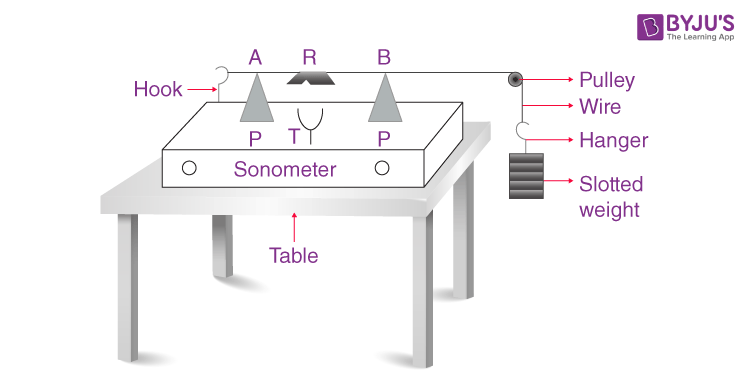# To Find The Frequency Of AC Mains With A Sonometer

## Aim

To determine the frequency of AC mains using a sonometer.

## Apparatus

• A sonometer with soft wires
• A set of eight tuning forks
• Seven ½ kg slotted weights
• A set of eight tuning forks
• Clamp
• Paper rider
• Metre Scale

## Diagram## Theory

Let us consider the alternating current to have a frequency v so that the frequency of magnetisation of the electromagnet VE becomes 2v.

Let a loaded stretched soft iron wire have a resonant length l1 with the electromagnet. Let a tuning fork of frequency VT have resonant length l2.

According to the law of length,

$$\begin{array}{l}v_{E}l_{1}=V_{r}l_{2}\end{array}$$

$$\begin{array}{l}v_{E}=V_{r}\frac{l_{2}}{l_{1}}\end{array}$$

Hence, the frequency of the alternating current is calculated using the formula

$$\begin{array}{l}v=\frac{1}{2}v_{E}=\frac{1}{2}v_{T}\frac{l_{2}}{l_{1}}\end{array}$$

The natural frequency of oscillation for a stretched wire of length L, mass M and tension T is given by

$$\begin{array}{l}n=\frac{1}{2L}\sqrt{\frac{T}{\mu }}\end{array}$$

$$\begin{array}{l}\mu=\frac{mass}{length}=\frac{m}{l}\end{array}$$

## Procedure

1. Place the sonometer on the table as shown in the figure.
2. Test the pulley and make it frictionless by oiling.
3. Put suitable weights in the hanger.
4. Move the wooden bridge P outward to include the maximum length of wire AB.
5. Decrease the length of the wire by moving the wooden bridges equally inwardly.
6. Go on decreasing the length till the sonometer wire starts vibrating.
7. The length of the wire can be adjusted for the maximum amplitude of vibration.
8. Measure the length of the wire AB between the edges of the two bridges and record it in length decreasing columns.
9. Bring the bridges closer and adjust the length for the maximum amplitude by increasing it.
10. Measure the length and record it in length increasing column.
11. Now take a tuning fork of minimum known frequency and adjust the wire length with the vibrating tuning fork.
12. Repeat step 11 above with tuning forks of other known frequencies.

## Observation

The weight suspended on the wire, T = _____ kg.

The length of the sonometer wire, L = _____ m.

Mass of wire, m = _____ kg.

Linear density,

$$\begin{array}{l}\mu =\frac{m}{L}\,kgm^{-1}\end{array}$$

 S. No Frequency of electromagnet and tuning fork used v(Hz) Resonant length of wire Frequency of electromagnet vE(Hz) $$\begin{array}{l}n=\frac{1}{2L}\sqrt{\frac{T}{\mu}}\end{array}$$ Length decreasing l’ (cm) Length increasing l”(cm) $$\begin{array}{l}Mean=\frac{l’+l”}{2}\end{array}$$ 1. 2. 3. 4. 5. 6. 7. vE 256 288 320 384 480 512 l1 = l2= l3= l4= l5= l6= l7= 256l2/l1= 288l3/l = 320l4/l = 384l5/l = 480l6/l = 512 l7/l =

Table for frequency and length

## Calculations

Using the formula, vE = v2l2/l1, calculate vE with observations 2 to 7.

Record these values in column 4 of the table.

Find the mean of the above six values of vE.

Then the frequency of the alternating current can be determined by the formula,

v = vE/2

Use

$$\begin{array}{l}n=\frac{1}{2L}\sqrt{\frac{T}{\mu }}\end{array}$$
for each observation and take the mean. Lastly, compare it with the standard frequency (50 Hz).

## Result

The frequency of the alternating current is _____ Hz.

Percentage Error

The actual frequency = 50 Hz

Difference = _____ Hz

$$\begin{array}{l}Percentage Error = \frac{Difference}{Actual Value}\times 100\, \%\end{array}$$

The error is within limits of experimental limits.

## Precaution

1. The wire should be soft iron or any other magnetic material.
2. Length should be noted when the amplitude of vibration is maximum.
3. Tip of the electromagnet should be very close to the wire in its middle

## Viva Voce

1. How does AC differ from DC?
2. Ans: DC has the same magnitude and direction throughout, while AC has to change magnitude and direction.

3. What is resonance?
4. Ans: When the natural frequency is equal to the frequency of the driving force, then resonance takes place. These vibrations are known as resonant vibrations.

5. Why is AC more dangerous than DC?
6. Ans: It is dangerous due to the following reasons:

1. AC attracts while DC repels

2. AC gives a huge and sudden shock that can be fatal.

7. What is the skin effect?
8. Ans: In an alternating current, the moving electrons tend to drift towards the conductor’s surface while moving along its axis. This tendency increases with the frequency of A.C in very high-frequency A.C. Electrons flow only on the conductor’s surface. This phenomenon is known as the skin effect.

9. When will the wire resonate?
10. Ans: When the frequency of A.C mains is equal to the natural frequency of the vibration of the wire.

Stay tuned with Byju’s to get the latest notification on CBSE, the CBSE syllabus, sample papers, marking scheme, and more.